# With-Abstraction¶

With abstraction was first introduced by Conor McBride [McBride2004] and lets you pattern match on the result of an intermediate computation by effectively adding an extra argument to the left-hand side of your function.

## Usage¶

In the simplest case the with construct can be used just to discriminate on the result of an intermediate computation. For instance

filter : {A : Set} → (A → Bool) → List A → List A
filter p [] = []
filter p (x ∷ xs) with p x
filter p (x ∷ xs)    | true  = x ∷ filter p xs
filter p (x ∷ xs)    | false = filter p xs


The clause containing the with-abstraction has no right-hand side. Instead it is followed by a number of clauses with an extra argument on the left, separated from the original arguments by a vertical bar (|).

When the original arguments are the same in the new clauses you can use the ... syntax:

filter : {A : Set} → (A → Bool) → List A → List A
filter p [] = []
filter p (x ∷ xs) with p x
...                  | true  = x ∷ filter p xs
...                  | false = filter p xs


In this case ... expands to filter p (x ∷ xs). There are three cases where you have to spell out the left-hand side:

• If you want to do further pattern matching on the original arguments.
• When the pattern matching on the intermediate result refines some of the other arguments (see Dot patterns).
• To disambiguate the clauses of nested with abstractions (see Nested with-abstractions below).

### Generalisation¶

The power of with-abstraction comes from the fact that the goal type and the type of the original arguments are generalised over the value of the scrutinee. See Technical details below for the details. This generalisation is important when you have to prove properties about functions defined using with. For instance, suppose we want to prove that the filter function above satisfies some property P. Starting out by pattern matching of the list we get the following (with the goal types shown in the holes)

postulate P : ∀ {A} → List A → Set
postulate p-nil : P []
postulate Q : Set
postulate q-nil : Q

proof : {A : Set} (p : A → Bool) (xs : List A) → P (filter p xs)
proof p []       = {! P [] !}
proof p (x ∷ xs) = {! P (filter p xs | p x) !}


In the cons case we have to prove that P holds for filter p xs | p x. This is the syntax for a stuck with-abstraction–filter cannot reduce since we don’t know the value of p x. This syntax is used for printing, but is not accepted as valid Agda code. Now if we with-abstract over p x, but don’t pattern match on the result we get:

proof : {A : Set} (p : A → Bool) (xs : List A) → P (filter p xs)
proof p [] = p-nil
proof p (x ∷ xs) with p x
...                 | r   = {! P (filter p xs | r) !}


Here the p x in the goal type has been replaced by the variable r introduced for the result of p x. If we pattern match on r the with-clauses can reduce, giving us:

proof : {A : Set} (p : A → Bool) (xs : List A) → P (filter p xs)
proof p [] = p-nil
proof p (x ∷ xs) with p x
...                 | true  = {! P (x ∷ filter p xs) !}
...                 | false = {! P (filter p xs) !}


Both the goal type and the types of the other arguments are generalised, so it works just as well if we have an argument whose type contains filter p xs.

proof₂ : {A : Set} (p : A → Bool) (xs : List A) → P (filter p xs) → Q
proof₂ p [] _ = q-nil
proof₂ p (x ∷ xs) H with p x
...                    | true  = {! H : P (filter p xs) !}
...                    | false = {! H : P (x ∷ filter p xs) !}


The generalisation is not limited to scrutinees in other with-abstractions. All occurrences of the term in the goal type and argument types will be generalised.

Note that this generalisation is not always type correct and may result in a (sometimes cryptic) type error. See Ill-typed with-abstractions below for more details.

### Nested with-abstractions¶

With-abstractions can be nested arbitrarily. The only thing to keep in mind in this case is that the ... syntax applies to the closest with-abstraction. For example, suppose you want to use ... in the definition below.

compare : Nat → Nat → Comparison
compare x y with x < y
compare x y    | false with y < x
compare x y    | false    | false = equal
compare x y    | false    | true  = greater
compare x y    | true = less


You might be tempted to replace compare x y with ... in all the with-clauses as follows.

compare : Nat → Nat → Comparison
compare x y with x < y
...            | false with y < x
...                       | false = equal
...                       | true  = greater
...            | true = less    -- WRONG


This, however, would be wrong. In the last clause the ... is interpreted as belonging to the inner with-abstraction (the whitespace is not taken into account) and thus expands to compare x y | false | true. In this case you have to spell out the left-hand side and write

compare : Nat → Nat → Comparison
compare x y with x < y
...            | false with y < x
...                       | false = equal
...                       | true  = greater
compare x y    | true = less


### Simultaneous abstraction¶

You can abstract over multiple terms in a single with abstraction. To do this you separate the terms with vertical bars (|).

compare : Nat → Nat → Comparison
compare x y with x < y | y < x
...            | true  | _     = less
...            | _     | true  = greater
...            | false | false = equal


In this example the order of abstracted terms does not matter, but in general it does. Specifically, the types of later terms are generalised over the values of earlier terms. For instance

postulate plus-commute : (a b : Nat) → a + b ≡ b + a
postulate P : Nat → Set

thm : (a b : Nat) → P (a + b) → P (b + a)
thm a b t with a + b | plus-commute a b
thm a b t    | ab    | eq = {! t : P ab, eq : ab ≡ b + a !}


Note that both the type of t and the type of the result eq of plus-commute a b have been generalised over a + b. If the terms in the with-abstraction were flipped around, this would not be the case. If we now pattern match on eq we get

thm : (a b : Nat) → P (a + b) → P (b + a)
thm a b t with   a + b  | plus-commute a b
thm a b t    | .(b + a) | refl = {! t : P (b + a) !}


and can thus fill the hole with t. In effect we used the commutativity proof to rewrite a + b to b + a in the type of t. This is such a useful thing to do that there is special syntax for it. See Rewrite below.

A limitation of generalisation is that only occurrences of the term that are visible at the time of the abstraction are generalised over, but more instances of the term may appear once you start filling in the right-hand side or do further matching on the left. For instance, consider the following contrived example where we need to match on the value of f n for the type of q to reduce, but we then want to apply q to a lemma that talks about f n:

postulate
R     : Set
P     : Nat → Set
f     : Nat → Nat
lemma : ∀ n → P (f n) → R

Q : Nat → Set
Q zero    = ⊥
Q (suc n) = P (suc n)

proof : (n : Nat) → Q (f n) → R
proof n q with f n
proof n ()   | zero
proof n q    | suc fn = {! q : P (suc fn) !}


Once we have generalised over f n we can no longer apply the lemma, which needs an argument of type P (f n). To solve this problem we can add the lemma to the with-abstraction:

proof : (n : Nat) → Q (f n) → R
proof n q with f n    | lemma n
proof n ()   | zero   | _
proof n q    | suc fn | lem = lem q


In this case the type of lemma n (P (f n) → R) is generalised over f n so in the right hand side of the last clause we have q : P (suc fn) and lem : P (suc fn) → R.

See The inspect idiom below for an alternative approach.

### Using underscores in pattern repetition¶

If an ellipsis ... cannot be used, the with-clause has to repeat (or refine) the patterns of the parent clause. Since Agda 2.5.3, such patterns can be replaced by underscores _ if the variables they bind are not needed. Here is a (slightly contrived) example:

record R : Set where
coinductive -- disallows matching
field  f  :  Bool
n  :  Nat

data P (r : R) : Nat → Set where
fTrue  :  R.f r ≡ true  →  P r zero
nSuc   :                   P r (suc (R.n r))

data Q : (b : Bool) (n : Nat) →  Set where
true!  :             Q true zero
suc!   :  ∀{b n}  →  Q b (suc n)

test : (r : R) {n : Nat} (p : P r n) → Q (R.f r) n
test  r  nSuc       = suc!
test  r  (fTrue p)  with  R.f r
test  _  (fTrue ())    |  false
test  _  _             |  true  = true!  -- underscore instead of (isTrue _)


### Rewrite¶

Remember example of simultaneous abstraction from above.

postulate plus-commute : (a b : Nat) → a + b ≡ b + a

thm : (a b : Nat) → P (a + b) → P (b + a)
thm a b t with   a + b  | plus-commute a b
thm a b t    | .(b + a) | refl = t


This pattern of rewriting by an equation by with-abstracting over it and its left-hand side is common enough that there is special syntax for it:

thm : (a b : Nat) → P (a + b) → P (b + a)
thm a b t rewrite plus-commute a b = t


The rewrite construction takes a term eq of type lhs ≡ rhs, where _≡_ is the built-in equality type, and expands to a with-abstraction of lhs and eq followed by a match of the result of eq against refl:

f ps rewrite eq = v

-->

f ps with lhs | eq
...    | .rhs | refl = v


One limitation of the rewrite construction is that you cannot do further pattern matching on the arguments after the rewrite, since everything happens in a single clause. You can however do with-abstractions after the rewrite. For instance,

postulate T : Nat → Set

isEven : Nat → Bool
isEven zero = true
isEven (suc zero) = false
isEven (suc (suc n)) = isEven n

thm₁ : (a b : Nat) → T (a + b) → T (b + a)
thm₁ a b t rewrite plus-commute a b with isEven a
thm₁ a b t | true  = t
thm₁ a b t | false = t


Note that the with-abstracted arguments introduced by the rewrite (lhs and eq) are not visible in the code.

### The inspect idiom¶

When you with-abstract a term t you lose the connection between t and the new argument representing its value. That’s fine as long as all instances of t that you care about get generalised by the abstraction, but as we saw above this is not always the case. In that example we used simultaneous abstraction to make sure that we did capture all the instances we needed. An alternative to that is to use the inspect idiom, which retains a proof that the original term is equal to its abstraction.

In the simplest form, the inspect idiom uses a singleton type:

data Singleton {a} {A : Set a} (x : A) : Set a where
_with≡_ : (y : A) → x ≡ y → Singleton x

inspect : ∀ {a} {A : Set a} (x : A) → Singleton x
inspect x = x with≡ refl


Now instead of with-abstracting t, you can abstract over inspect t. For instance,

filter : {A : Set} → (A → Bool) → List A → List A
filter p [] = []
filter p (x ∷ xs) with inspect (p x)
...                  | true  with≡ eq = {! eq : p x ≡ true !}
...                  | false with≡ eq = {! eq : p x ≡ false !}


Here we get proofs that p x ≡ true and p x ≡ false in the respective branches that we can on use the right. Note that since the with-abstraction is over inspect (p x) rather than p x, the goal and argument types are no longer generalised over p x. To fix that we can replace the singleton type by a function graph type as follows (see Anonymous modules to learn about the use of a module to bind the type arguments to Graph and inspect):

module _ {a b} {A : Set a} {B : A → Set b} where

data Graph (f : ∀ x → B x) (x : A) (y : B x) : Set b where
ingraph : f x ≡ y → Graph f x y

inspect : (f : ∀ x → B x) (x : A) → Graph f x (f x)
inspect _ _ = ingraph refl


To use this on a term g v you with-abstract over both g v and inspect g v. For instance, applying this to the example from above we get

postulate
R     : Set
P     : Nat → Set
f     : Nat → Nat
lemma : ∀ n → P (f n) → R

Q : Nat → Set
Q zero    = ⊥
Q (suc n) = P (suc n)

proof : (n : Nat) → Q (f n) → R
proof n q with f n    | inspect f n
proof n ()   | zero   | _
proof n q    | suc fn | ingraph eq = {! q : P (suc fn), eq : f n ≡ suc fn !}


We could then use the proof that f n ≡ suc fn to apply lemma to q.

This version of the inspect idiom is defined (using slightly different names) in the standard library in the module Relation.Binary.PropositionalEquality and in the agda-prelude in Prelude.Equality.Inspect (reexported by Prelude).

### Alternatives to with-abstraction¶

Although with-abstraction is very powerful there are cases where you cannot or don’t want to use it. For instance, you cannot use with-abstraction if you are inside an expression in a right-hand side. In that case there are a couple of alternatives.

#### Pattern lambdas¶

Agda does not have a primitive case construct, but one can be emulated using pattern matching lambdas. First you define a function case_of_ as follows:

case_of_ : ∀ {a b} {A : Set a} {B : Set b} → A → (A → B) → B
case x of f = f x


You can then use this function with a pattern matching lambda as the second argument to get a Haskell-style case expression:

filter : {A : Set} → (A → Bool) → List A → List A
filter p [] = []
filter p (x ∷ xs) =
case p x of
λ { true  → x ∷ filter p xs
; false → filter p xs
}


This version of case_of_ only works for non-dependent functions. For dependent functions the target type will in most cases not be inferrable, but you can use a variant with an explicit B for this case:

case_return_of_ : ∀ {a b} {A : Set a} (x : A) (B : A → Set b) → (∀ x → B x) → B x
case x return B of f = f x


The dependent version will let you generalise over the scrutinee, just like a with-abstraction, but you have to do it manually. Two things that it will not let you do is

• further pattern matching on arguments on the left-hand side, and
• refine arguments on the left by the patterns in the case expression. For instance if you matched on a Vec A n the n would be refined by the nil and cons patterns.

#### Helper functions¶

Internally with-abstractions are translated to auxiliary functions (see Technical details below) and you can always write these functions manually. The downside is that the type signature for the helper function needs to be written out explicitly, but fortunately the Emacs Mode has a command (C-c C-h) to generate it using the same algorithm that generates the type of a with-function.

### Performance considerations¶

The generalisation step of a with-abstraction needs to normalise the scrutinee and the goal and argument types to make sure that all instances of the scrutinee are generalised. The generalisation also needs to be type checked to make sure that it’s not ill-typed. This makes it expensive to type check a with-abstraction if

• the normalisation is expensive,
• the normalised form of the goal and argument types are big, making finding the instances of the scrutinee expensive,
• type checking the generalisation is expensive, because the types are big, or because checking them involves heavy computation.

In these cases it is worth looking at the alternatives to with-abstraction from above.

## Technical details¶

Internally with-abstractions are translated to auxiliary functions–there are no with-abstractions in the Core language. This translation proceeds as follows. Given a with-abstraction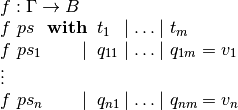where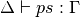(i.e.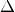types the variables bound in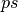), we

• Infer the types of the scrutinees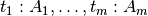.

• Partition the contextinto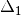and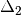such thatis the smallest context where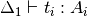for all, i.e., where the scrutinees are well-typed. Note that the partitioning is not required to be a split,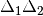can be a (well-formed) reordering of.

• Generalise over the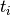s, by computing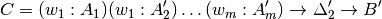such that the normal form of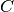does not contain anyand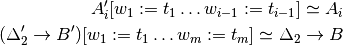where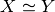is equality of the normal forms of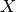and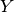. The type of the auxiliary function is then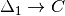.

• Check thatis type correct, which is not guaranteed (see below).

• Add a function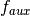, mutually recursive with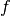, with the definition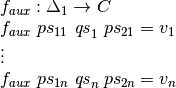where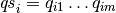, and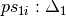and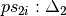are the patterns from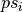corresponding to the variables of. Note that due to the possible reordering of the partitioning ofintoand, the patterns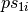and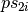can be in a different order from how they appear.

• Replace the with-abstraction by a call toresulting in the final definition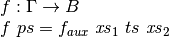where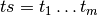and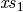and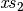are the variables fromcorresponding toandrespectively.

### Examples¶

Below are some examples of with-abstractions and their translations.

postulate
A     : Set
_+_   : A → A → A
T     : A → Set
mkT   : ∀ x → T x
P     : ∀ x → T x → Set

-- the type A of the with argument has no free variables, so the with
-- argument will come first
f₁ : (x y : A) (t : T (x + y)) → T (x + y)
f₁ x y t with x + y
f₁ x y t    | w = {!!}

-- Generated with function
f-aux₁ : (w : A) (x y : A) (t : T w) → T w
f-aux₁ w x y t = {!!}

-- x and p are not needed to type the with argument, so the context
-- is reordered with only y before the with argument
f₂ : (x y : A) (p : P y (mkT y)) → P y (mkT y)
f₂ x y p with mkT y
f₂ x y p    | w = {!!}

f-aux₂ : (y : A) (w : T y) (x : A) (p : P y w) → P y w
f-aux₂ y w x p = {!!}

postulate
H : ∀ x y → T (x + y) → Set

-- Multiple with arguments are always inserted together, so in this case
-- t ends up on the left since it’s needed to type h and thus x + y isn’t
-- abstracted from the type of t
f₃ : (x y : A) (t : T (x + y)) (h : H x y t) → T (x + y)
f₃ x y t h with x + y | h
f₃ x y t h    | w₁    | w₂ = {! t : T (x + y), goal : T w₁ !}

f-aux₃ : (x y : A) (t : T (x + y)) (h : H x y t) (w₁ : A) (w₂ : H x y t) → T w₁
f-aux₃ x y t h w₁ w₂ = {!!}

-- But earlier with arguments are abstracted from the types of later ones
f₄ : (x y : A) (t : T (x + y)) → T (x + y)
f₄ x y t with x + y | t
f₄ x y t    | w₁    | w₂ = {! t : T (x + y), w₂ : T w₁, goal : T w₁ !}

f-aux₄ : (x y : A) (t : T (x + y)) (w₁ : A) (w₂ : T w₁) → T w₁
f-aux₄ x y t w₁ w₂ = {!!}


### Ill-typed with-abstractions¶

As mentioned above, generalisation does not always produce well-typed results. This happens when you abstract over a term that appears in the type of a subterm of the goal or argument types. The simplest example is abstracting over the first component of a dependent pair. For instance,

postulate
A : Set
B : A → Set
H : (x : A) → B x → Set

bad-with : (p : Σ A B) → H (fst p) (snd p)
...           | _ = {!!}


Here, generalising over fst p results in an ill-typed application H w (snd p) and you get the following type error:

fst p != w of type A
when checking that the type (p : Σ A B) (w : A) → H w (snd p) of
the generated with function is well-formed


This message can be a little difficult to interpret since it only prints the immediate problem (fst p != w) and the full type of the with-function. To get a more informative error, pointing to the location in the type where the error is, you can copy and paste the with-function type from the error message and try to type check it separately.

  The termination checker has special treatment for with-functions, so replacing a with by the equivalent helper function might fail termination.
 [McBride2004] C. McBride and J. McKinna. The view from the left. Journal of Functional Programming, 2004. http://strictlypositive.org/vfl.pdf.